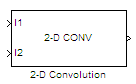Main Content

# 2-D Convolution

Compute 2-D discrete convolution of two input matrices

• Library:
• Computer Vision Toolbox / Filtering

•## Description

The 2-D Convolution block computes the two-dimensional convolution of two input matrices. Assume that matrix A has dimensions (Ma, Na) and matrix B has dimensions (Mb, Nb). When the block calculates the full output size, the equation for the 2-D discrete convolution is:

`$C\left(i,j\right)=\sum _{m=0}^{\left(Ma-1\right)}\sum _{n=0}^{\left(Na-1\right)}A\left(m,n\right)*B\left(i-m,j-n\right)$`

where $0\le i and $0\le j.

## Ports

### Input

expand all

Input matrix, specified as either a matrix of intensity values or a matrix that represents one plane of an RGB video stream.

Data Types: `single` | `double` | `int8` | `int16` | `int32` | `int64` | `uint8` | `uint16` | `uint32` | `uint64` | `fixed point`

Input matrix, specified as either a matrix of intensity values or a matrix that represents one plane of an RGB video stream.

Data Types: `single` | `double` | `int8` | `int16` | `int32` | `int64` | `uint8` | `uint16` | `uint32` | `uint64` | `fixed point`

### Output

expand all

Convolution of the input matrices, returned as a matrix.

#### Dependencies

• The dimensions of the output are dictated by the Output size parameter.

• If the data type of the input is floating point, the output of the block is also floating point.

• If `all(size(I1)<size(I2))`, the block returns an error.

Data Types: `single` | `double` | `int8` | `int16` | `int32` | `int64` | `uint8` | `uint16` | `uint32` | `uint64` | `fixed point`

## Parameters

expand all

Main Tab

Dimensions of the output.

The table describes the block output, given the following input dimensions:

• I1 — (Ma, Na)

• I2 — (Mb, Nb)

Output sizeOutput Output Dimension
`Full`Full two-dimensional convolution(Ma+Mb-1, Na+Nb-1).
`Same as input port I1`Central part of the convolution with the same dimensions as the input at port I1
`Valid`Only the parts of the convolution that are computed without the zero-padded edges of any input.(Ma-Mb+1, Na-Nb+1)

Normalize output by dividing the output by `sqrt(sum(dot(I1p,I1p))*sum(dot(I2,I2)))`, where `I1p` is the portion of the I1 matrix that aligns with the I2 matrix.

Note

When you select the Normalized output check box, the block input cannot be fixed point.

Data Types Tab

For details on the fixed-point block parameters, see Specify Fixed-Point Attributes for Blocks .

## Block Characteristics

 Data Types `double` | `fixed point` | `integer` | `single` Multidimensional Signals `no` Variable-Size Signals `yes`

expand all

## See Also

Introduced before R2006a

Download ebook